# SAT Math Multiple Choice Question 352: Answer and Explanation

### Test Information

Question: 352

7.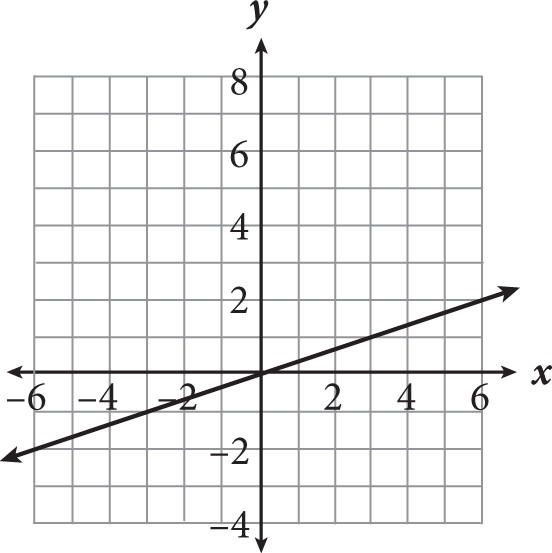If the equation of the line shown in the figure above is written in the form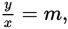which of the following could be the value of m?

• A. -3
• B.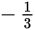• C.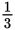• D. 3

Getting to the Answer: Because b is 0, the equation of this line in slope-intercept form is y = mx, which can be rewritten as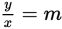. Count the rise and the run from the origin, (0, 0), to the next point, (3, 1), to get a slope of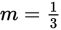.